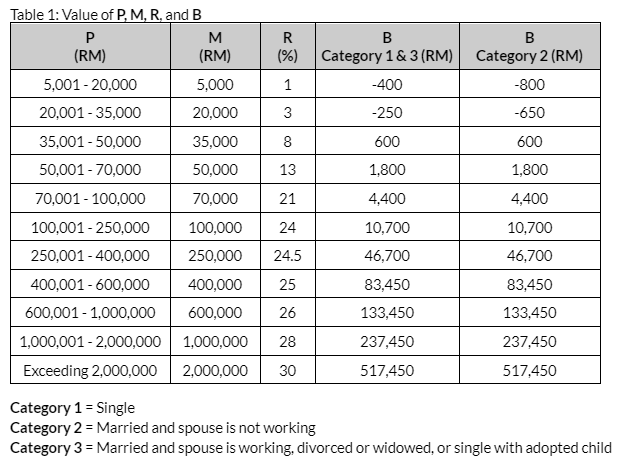In this article, we will look at an example of calculating PCB/MTD. PCB stands for Potongan Cukai Bulanan in Malay as known as MTD(Monthly Tax Deduction). It involves 4 steps.

Step 1: Retrieve employee info

Step 2: Get the employee's past payroll records/previous employment records

Step 3: Get the current month's payroll details

Step 4: Identify the type of PCB computerized calculation

Example

PCB for Feb 2022. Below is detailed information about Mr. Lim.

• He is a Malaysian citizen.
• He is married and his spouse is working.
• He has 2 children under the age of 18
• His gross monthly salary is RM5,000.
• He is entitled to a monthly fixed allowance of RM200. It is tax exempted.

Step 1: Retrieve employee info

• Residence status: Resident
• Marital Status: Married
• Disabled Individual: No
• Spouse Working: Yes
• Disabled Spouse: No
• Number of Children: 2 children under the age of 18
• Worker Type: Normal

Step 2: Get the employee's past payroll/previous employment records

ItemAmount(RM)
Accumulated PCB paid
16.65
Accumulated income from previous month
5,000
Accumulated EPF
550
Accumulated tax relief deduction
0
Accumulated Zakat paid
0

Step 3: Get the current month's payroll details

ItemAmount(RM)

Monthly wage

5,000

EPF contribution

550(with a rate of 11%)

2,500

Fixed allowance

200 (Exempted)

Zakat0
Payroll monthFebruary

Step 4: Identify the type of PCB computerized calculation

It belongs to PCB computerized calculation for Normal Remuneration.

PCB for current month =  [ [(P – M) x R + B] – (Z+ X)] ] / (n+1)

There are 4 steps to calculating PCB using computerized calculation

4(A) Determine category of employee

4(B) Determine chargeable income for a year [P]

4(C) Determine monthly MTD for net normal remuneration

4(D) Calculate the Net PCB

4(A) Determine category of employee

Category 3, since Mr. Lim is married and his spouse is working.

4(B) Determine chargeable income for a year [P]

i) Firstly, determine the value of K2

K2

=  Estimated balance of total contribution to EPF or other Approved Scheme for the balance of the qualifying month

Where n = 10(as we count the MTD for Feb)

= [ [4000.00(max limit) - (K + K1 + Kt)] / n ] or K1, whichever is lower

= [ [4000.00 - (550.00 + 550.00 + 0.00)] / 10 ]

=290.00

K1 is RM550 which is higher than the estimated(290.00)So, K2 is RM290.00.

ii) Secondly, determine num of qualifying children(Q).

2 children below age 18 => entitled to 2

Q= 2

iii) Finally, calculate the value of P.

P =  [ ∑(Y – K) + (Y1 – K1) + [(Y2 – K2) n ] ] –  [ D + S + DU + SU + QC + (∑LP + LP1) ]

= [ ∑(5000.00 – 550.00) + (5000.00 – 550.00) + [(5000 – 290.00) x 10 ] ] –  [9000.00 + 0.00 + 0.00 + 0.00 + 2000.00(2) + (0.00 + 2500.00) ]

= 40500.00

4(C) Determine monthly MTD for net normal remunerationUpon getting the value of P, the value of MR, and B are determined based on Table 1.

P40500.00

So M= 35000, R = 8%, B = 600 (Employee belongs to Category 3)

PCB for current month

= [ [(P – M) x R + B] – (Z+ X)] ] / (n+1)

[(40500.00 - 35000.00) x 0.08 - 600.00] - (0.00 + 16.65)] / (10+1)

= 93.03

Check the rule: PCB before zakat deduction below RM10 should reflect RM0. Otherwise, deduct as per PCB calculation. Hence, PCB for the current month is RM93.03.

4(D) Calculate the Net PCB

Net PCB

PCB for current month – Zakat/Fi/Levi for current month

= 93.03 - 0

=93.03

Check the rule: PCB after zakat deduction below RM10 should reflect RM0. Otherwise, deduct as per PCB calculation. Hence, Net PCB is RM93.03.

Finally, rounded up the amount to the nearest 5 cents.

Net PCB is RM93.05